#### Geometric data analyis, beyond convolutions

I defended my PhD thesis on July 2nd, 2020: a related video recording is available here. My thesis is the most up-to-date reference on my work: it is written as an introductory textbook of data sciences and shape analysis, from a geometric perspective. If you just saw one of my talks on symbolic matrices and large-scale optimal transport, here is what you are looking for: KeOps library, GeomLoss package, Slides for the defense, PhD thesis.

#### KeOps : Kernel Operations on the GPU, with autodiff, without memory overflows

##### Since September 2017

Automatic differentiation is a game changer for applied mathematicians: thanks to modern python libraries (PyTorch, TensorFlow), gradients of arbitrary loss functions can now be computed effortlessly. Unfortunately though, major frameworks keep a strong focus on Convolutional Neural Networks and do not support well operations on point clouds: relying on tensorized matrix-centric computations, their core routines have huge memory footprints that prevent users from working with large meshes or random samples.

As an example, let's say that $(x_i)\in\mathbb{R}^{\text{N}\times\text{D}}$ and $(y_j)\in\mathbb{R}^{\text{M}\times\text{D}}$ are clouds of $\text{N}$ and $\text{M}$ points in $\mathbb{R}^\text{D}$. To compute a quantity such as $$f(x_i)~=~\frac{1}{\text{M}}\sum_{j=1}^\text{M} \exp(-\|x_i-y_j\|^2/2\sigma^2)$$ for all $i$ in $[1,\text{N}]$, a PyTorch, NumPy or Matlab user would typically compute a kernel matrix $$K_{i,j}~=~ \exp(-\|x_i-y_j\|^2/2\sigma^2)$$ before applying a line-wise sum reduction. Problem is: such a matrix $K$ does not fit contiguously in the GPU memory as soon as $\sqrt{NM}$ exceeds, say, $10,000$, and users get frustrated by a memory overflow error:

RuntimeError: cuda runtime error (2) : out of memory at /opt/conda/.../THCStorage.cu:66

Developed by Benjamin Charlier, Joan Glaunès and myself, the KeOps library breaks this memory bottleneck without compromising on usability. Coming with Matlab, NumPy and PyTorch bindings, our library lets you compute arbitrary expressions of the form $$\text{Out}_i~=~ \text{Reduction}_{j=1}^\text{M}\big[ \text{Formula}( P^1, P^2, \dots, X_i^1, X_i^2, \dots, Y_j^1, Y_j^2, \dots) \big]$$ and the associated gradients (of arbitrary order), for arbitrary values of $\text{M}$ and $\text{N}$ : we guarantee a linear memory footprint with respect to your input data. Here:

• $\text{Out}_i$ is an output tensor indexed by $i$.
• $\text{Reduction}$ is a Sum, LogSumExp, Max, Min, ArgMax or ArgMin reduction.
• $\text{Formula}$ is a symbolic operation defined from a list of mathematical primitives.
• $P^1$, $P^2$, $\dots$ are vector parameters.
• $X^1_i$, $X^2_i$, $\dots$ are vector variables that depend on the output index $i$.
• $Y^1_j$, $Y^2_j$, $\dots$ are vector variables that depend on the reduction index $j$.

Under the hood, KeOps combines a tiled reduction scheme (written in CUDA) with a symbolic differentation engine implemented within C++11's templating system; from the user's point of view, it can be used as simply as any other PyTorch operation. To get started, here is a collection of useful links:

#### Global divergences between measures

##### Since December 2016

Aside from my PhD Thesis with Alain Trouvé, I have an ongoing collaboration with Gabriel Peyré, François-Xavier Vialard and Benjamin Charlier: we are investigating the use of Optimal Transport to drive measure-fitting pipelines.

With applications ranging from shape analysis to machine learning, we focus on the unbiased Sinkhorn divergence between measures to define a positive definite, convex and symmetric loss function. Our reference implementation scales up to millions of samples and allows users to retrieve well-behaved gradients with strong theoretical guarantees.

#### Steerable Wavelets for Medical Image Denoising

##### Summer 2015

My master's thesis was written during a 5-months long internship at Siemens CT/Healthcare in Princeton, NJ. I attempted to build on the work of Michael Unser and Nicolas Chenouard on steerable wavelets to improve the real-time denoising pipeline used in Siemens scanners and fluoroscopic devices.

Unfortunately, I signed a non-disclosure agreement which prevents me from putting my master's thesis online : as a consolation, here is a short "Introduction to a Research Topic" written to introduce my fellow ENS classmates to medical image denoising - in french.

#### Screened Poisson Surface Reconstruction

##### March 2015

In order to complete the Points Clouds and 3D Modeling MVA course by François Goulette, Jean-Emmanuel Deschaud and Tamy Boubekeur, I worked on the Screened Poisson surface reconstruction algorithm proposed in 2013 by Michael Kazhdan and Hugues Hoppe, eventually implementing it from scratch in the 2D euclidean plane.

My report can be found here, as well as the Matlab code and the beamer presentation.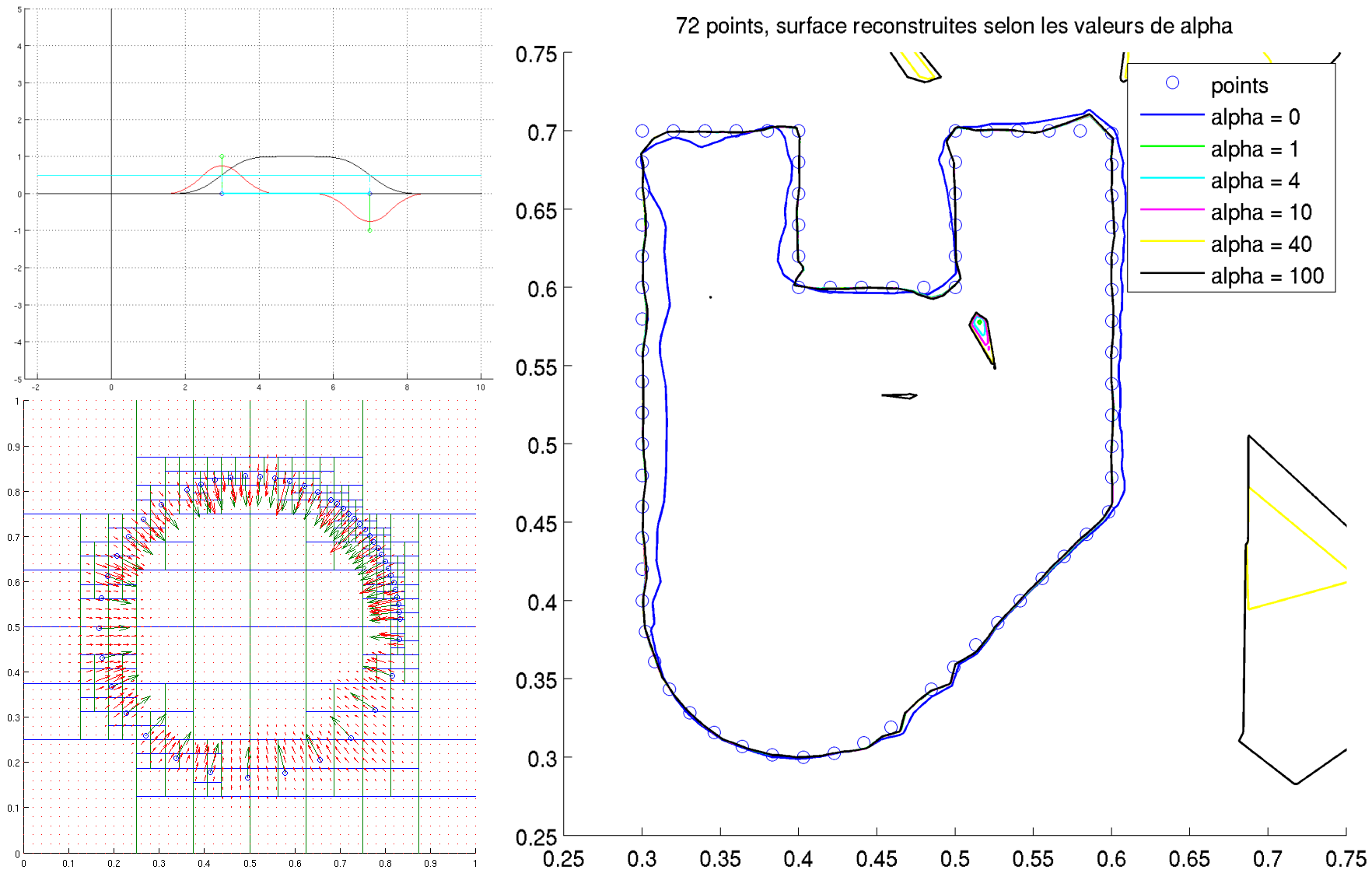#### Gradient Lines Drawing

##### January-February 2015

This is the project Vincent Vidal and I worked on for our MVA course : Sub-pixel Image Processing, by Lionel Moisan. Left free to design our own experiments and methods, Vincent and I wrote a comparative study on the methods to compute and visualize the gradient lines of an image.

Our report can be found here, as well as the code.#### SIFT Flow : Find the Difference !

##### January 2015

This is an project I worked on with Vincent Vidal, for the MVA course : Object Recognition and Computer Vision, by Jean Ponce, Ivan Laptev, Cordelia Schmid and Josef Sivic. Using the EECV 2008 SIFT Flow algorithm, we designed a way to compare two pictures of the same building detecting occlusions, deformations and drawing errors regardless of variations in graphic style.

Our report can be found here, as well as the code.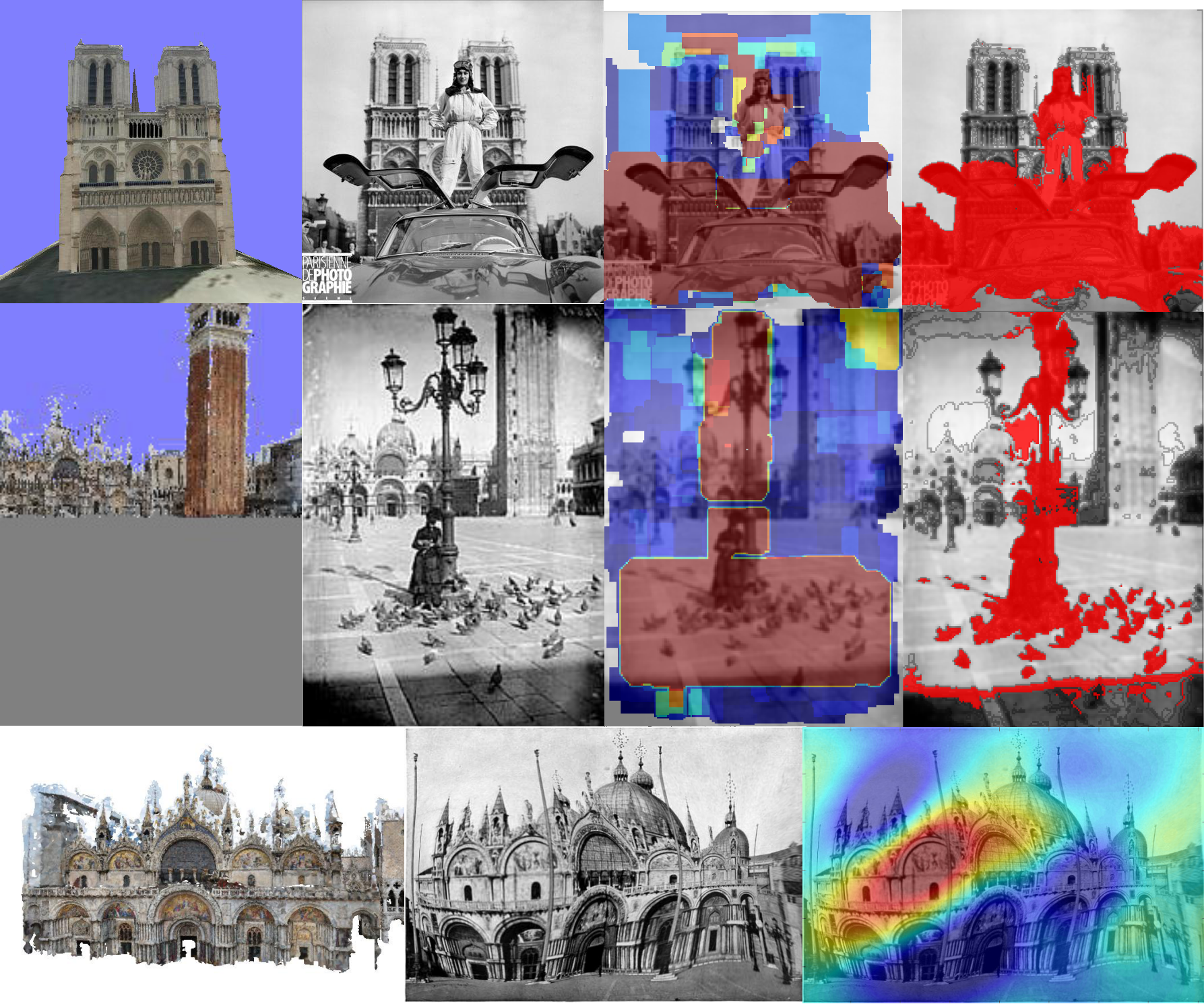#### Vocoder

##### May 2013

As part of my signal processing course at the École Normale Supérieure, Li-Yao Xia and I wrote a "Vocoder" using Matlab, a program which is able to modify the speed rate of a sound file without altering its pitch, following a method given in this paper by Michael R. Portnoff. A complete analysis of our results can be found here (in French). I deeply enjoyed "debugging" this program, testing and comparating various convolution windows.

Just a little example of what we achieved : a short excerpt from this video, followed by a speeded up version :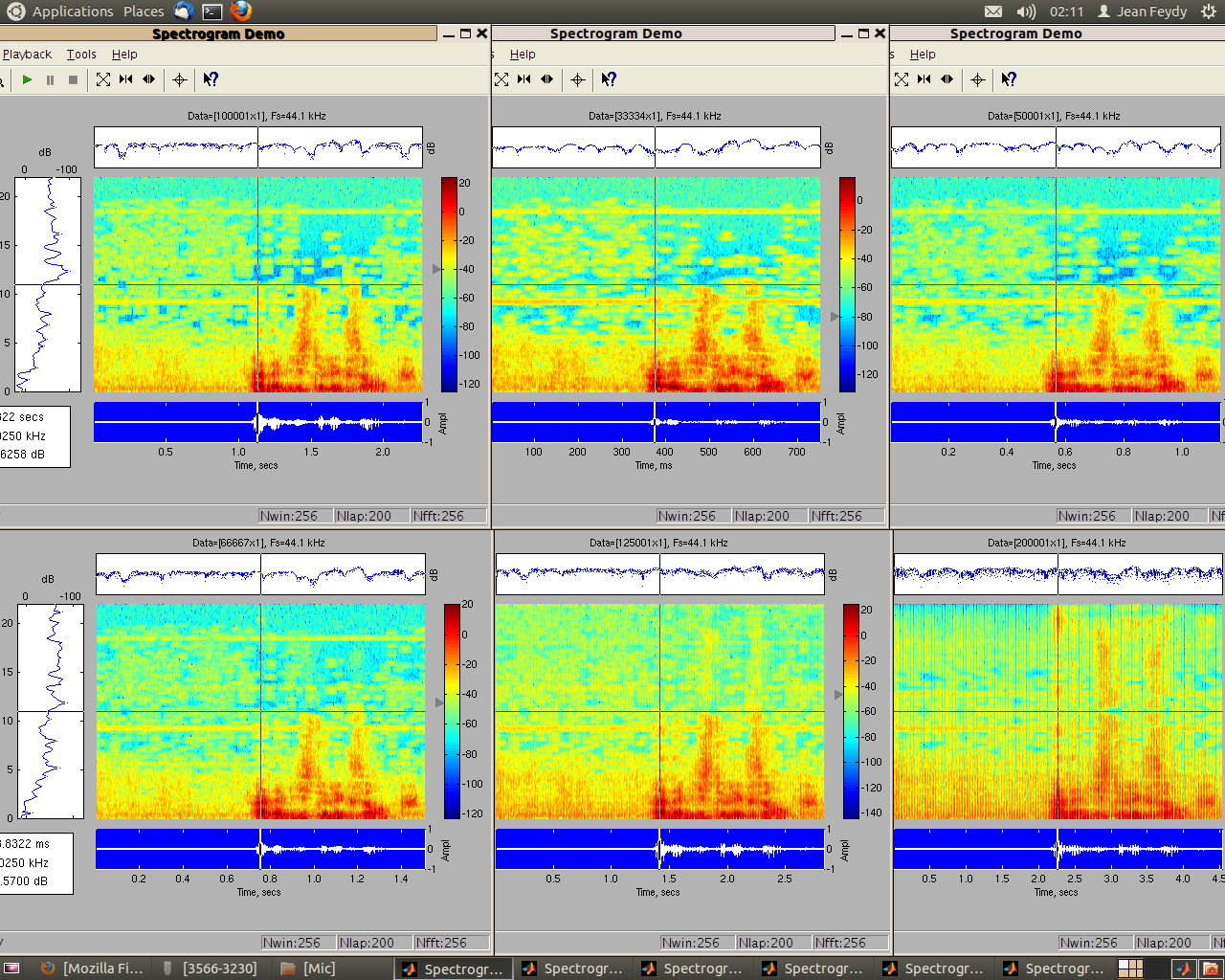#### MiniC compiler

##### December 2012

As part of my compilers course at the École Normale Supérieure, Ken Chanseau Saint-germain and I wrote in OCaml a MiniC (fragment of C) to MIPS assembly compiler. I found it extremely pleasant to understand the underlying constraints of programming languages such as C (for instance, I eventually understood how recursive functions or multiple inheritance were actually implemented).

Eventually, our MiniC compiler was able to compile and execute this Mandelbrot set drawing program, resulting in this MIPS assembly code, and the following output :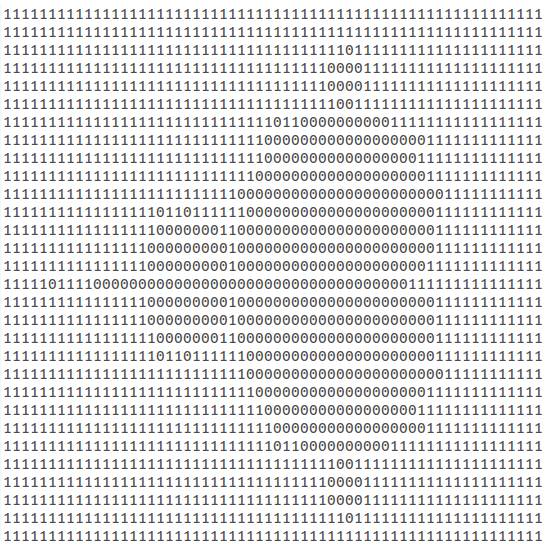#### Maths Courses to Trees

##### 2011-2013

Inspired by infographics books and innovative works such as Oliver Byrne's "The Elements of Euclid" (a must-have for every mathematician), I decided a few years ago that I would one day publish a mathematics handbook suiting my own taste. A first step would be to turn a Latex course into a mindmap.

As of today, I have coded a little software that turns an XML version of the course into a good-looking "inferencial" tree, using Graphviz, PGF/Tikz and dot2tex. Further versions will include a Latex to XML module (which will use the structure of the Latex file to infer logical links between theorems). I am currently working on a HTML5 interface, using SigmaJS, Perl and LaTeXML.

By way of example, here is a tree I actually used for my studies :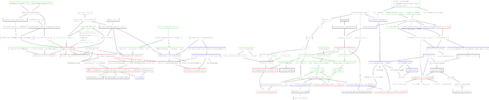#### Random and Discretized Mixing Maps

##### 2012-2013

As part of my studies at the École Normale Supérieure, Alexis Gilles and I wrote a memoir which is entitled "Random Permutations and Discretization of Mixing Maps" : Does the sampling of a mixing map produce a generic map ? Is it possible to infer properties of the continuous map from the discretized one ?

The paper features theorems and analysis of the statistics I computed (available here), our theoretical framework being the reduction modulo p of a polynomial with integer coefficients.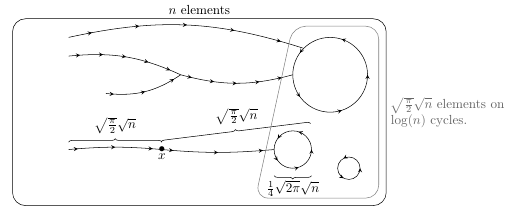#### Hyperbolic groups are Automatic

##### 2011

As a preparation to the competitive exam for entry to the École Normale Supérieure, I wrote a memoir on a subject at the border between geometric groups theory and computer science : Automatic and Hyperbolic groups. In a nutshell, considering a finitely generated group and its Cayley graph, we prove that if triangles in the graph are "slim" in Gromov sense, then the structure of the graph can somehow be described with regular expressions.

Here is a link to a summarized version of the work I did. By way of experiments, using this little OCaml program I coded and Graphviz, I computed several dozens of Cayley graphs (see below a few of them). I will upload slides as soon as possible.